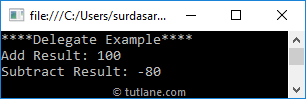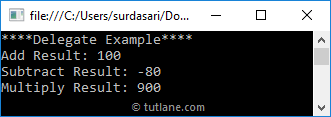# Visual Basic Delegates

In visual basic, the delegate is a type that defines a method signature and it is useful to hold the reference of one or more methods that is having the same signatures.

By using delegates, we can invoke the methods and send methods as an argument to other methods.

In visual basic, the delegate is a reference type and it’s type-safe and secure. The delegates are similar to function pointers in c++.

## Visual Basic Delegate Declaration Syntax

In visual basic, the declaration of delegate will be same as method signature but the only difference is we will use a `Delegate` keyword to define delegates.

Following is the syntax of defining a delegate using `Delegate` keyword in a visual basic programming language.

<access_modifier> Delegate <return_type> <delegate_name>(<parameters>)

Following is the example of declaring a delegate using `Delegate` keyword in visual basic.

Public Delegate Sub UserDetails(ByVal name As String);

If you observe the above example, the declaration of a delegate is same as method declaration with required parameter types and return value.

The above-defined method “UserDetails” can be pointed to any other method which is having the same parameters and return type.

In visual basic, the delegates must be instantiated with a method or an expression that has the same return type and parameters and we can invoke method through the delegate instance.

## Visual Basic Delegate Example

Following is the example of declaring and using a Delegate in a visual basic programming language.

Module Module1

' Declare Delegate

Public Delegate Sub SampleDelegate(ByVal a As Integer, ByVal b As Integer)

Class MathOperations

Public Sub Add(ByVal a As Integer, ByVal b As Integer)

Console.WriteLine("Add Result: {0}", a + b)

End Sub

Public Sub Subtract(ByVal x As Integer, ByVal y As Integer)

Console.WriteLine("Subtract Result: {0}", x - y)

End Sub

End Class

Sub Main(ByVal args As String())

Console.WriteLine("****Delegate Example****")

Dim m As MathOperations = New MathOperations()

' Instantiate delegate with add method

dlgt(10, 90)

' Instantiate delegate with subtract method

dlgt(10, 90)

End Sub

End Module

If you observe the above example, we created a delegate called “SampleDelegate” and the delegate has been instantiated by using defined methods.

When we execute the above visual basic program, we will get the result as shown below.This is how we can use delegates in our applications to call all the methods which are having the same signatures with a single object.

In visual basic, we can invoke the delegates same like a method or by using the Invoke method like as shown below.

dlgt(10, 90);

// or

dlgt.Invoke(10, 90);

## Visual Basic Delegate Types

In visual basic, we have a two different type of delegates available, those are

• Single cast Delegates
• Multicast Delegates

## Visual Basic Single Cast Delegates

In visual basic, a delegate which points to a single method is called a single cast delegate and it is useful to hold the reference of a single method like as explained in the above example.

## Visual Basic Multicast Delegates

In visual basic, a delegate that points to multiple methods is called a multicast delegate and it is useful to hold the reference of multiple methods with a single delegate.

By using `"+"` operator, we can add the multiple method references to the delegate object. Same way, by using `"-"` operator we can remove the method references from the delegate object.

In visual basic, Multicast delegates will work with only the methods which are having a `Sub` as return type. In case, if we want to create a multicast delegate with the return type, then we will get a return type of the last method in the invoking list.

## Visual Basic Multicast Delegate Example

Following is the example of implementing a multicast delegate to hold the reference of multiple methods with `"+"` operator in a visual basic programming language.

Module Module1

' Declare Delegate

Public Delegate Sub SampleDelegate(ByVal a As Integer, ByVal b As Integer)

Class MathOperations

Public Sub Add(ByVal a As Integer, ByVal b As Integer)

Console.WriteLine("Add Result: {0}", a + b)

End Sub

Public Sub Subtract(ByVal x As Integer, ByVal y As Integer)

Console.WriteLine("Subtract Result: {0}", x - y)

End Sub

Public Sub Multiply(ByVal x As Integer, ByVal y As Integer)

Console.WriteLine("Multiply Result: {0}", x * y)

End Sub

End Class

Sub Main(ByVal args As String())

Console.WriteLine("****Delegate Example****")

Dim m As MathOperations = New MathOperations()

Dim t2 As SampleDelegate = AddressOf m.Subtract

Dim t3 As SampleDelegate = AddressOf m.Multiply

Dim dlgt As SampleDelegate = MulticastDelegate.Combine(t1, t2, t3)

dlgt(10, 90)

End Sub

End Module

If you observe the above example, we created a delegate called “SampleDelegate” and holding the reference of multiple methods using `"+"` operator. Now, our delegate becomes a multicast delegate and invoking dlgt instance will invoke all the methods sequentially.

When we execute the above visual basic program, we will get the result as shown below.This is how we can use multicast delegates to hold the reference of multiple methods based on our requirements.

## Visual Basic Pass Method as Parameter using Delegate

In visual basic, by using delegate we can pass methods as a parameter. Following is the example of sending methods as a parameter using a delegate.

Module Module1

' Declare Delegate

Public Delegate Sub SampleDelegate(ByVal a As Integer, ByVal b As Integer)

Class MathOperations

Public Sub Add(ByVal a As Integer, ByVal b As Integer)

Console.WriteLine("Add Result: {0}", a + b)

End Sub

Public Sub Subtract(ByVal x As Integer, ByVal y As Integer)

Console.WriteLine("Subtract Result: {0}", x - y)

End Sub

Public Sub Multiply(ByVal x As Integer, ByVal y As Integer)

Console.WriteLine("Multiply Result: {0}", x * y)

End Sub

End Class

Sub Main(ByVal args As String())

Console.WriteLine("****Delegate Example****")

Dim m As MathOperations = New MathOperations()

End Sub

Private Sub SampleMethod(ByVal dlgt As SampleDelegate, ByVal a As Integer, ByVal b As Integer)

dlgt(a, b)

End Sub

End Module

If you observe the above example, we created a “SampleMethod” with delegate as a parameter type. By declaring like this, we can pass a method as a parameter to the newly created method (SampleMethod).

When we execute the above visual basic program, we will get the result as shown below.## Visual Basic Delegates Overview

The following are the important properties of delegate in a visual basic programming language.

• We need to use a `Delegate` keyword to define delegates.
• In visual basic, delegates are useful to hold the reference of one or more methods which are having the same signature as delegate.
• In visual basic, delegates are like function pointers in C++ but these are type-safe and secure.
• By using delegates, we can pass methods as a parameter to the other methods.
• In visual basic, we can invoke delegates as normal methods or by using Invoke property.
• By using delegates, we can call multiple methods with a single event.